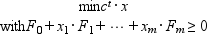Scilab Home page | Wiki | Bug tracker | Forge | Mailing list archives | ATOMS | File exchange
Please login or create an account
Change language to: English - Français - Português -

Please note that the recommended version of Scilab is 6.1.0. This page might be outdated.
See the recommended documentation of this function

Scilab help >> Optimization and Simulation > semidef

# semidef

semidefinite programming

### Calling Sequence

`[x,Z,ul,info]=semidef(x0,Z0,F,blck_szs,c,options)`

### Arguments

x0

m x 1 real column vector (must be strictly primal feasible, see below)

Z0

L x 1 real vector (compressed form of a strictly feasible dual matrix, see below)

F

L x (m+1) real matrix

blck_szs

p x 2 integer matrix (sizes of the blocks) defining the dimensions of the (square) diagonal blocks `size(Fi(j)=blck_szs(j) j=1,...,m+1`.

c

m x 1 real vector

options

row vector with five entries `[nu,abstol,reltol,0,maxiters]`

ul

row vector with two entries

### Description

`[x,Z,ul,info]=semidef(x0,Z0,F,blck_szs,c,options)` solves semidefinite program:and its dual:exploiting block structure in the matrices `F_i`.

It interfaces L. Vandenberghe and S. Boyd sp.c program.

The `Fi's` matrices are stored columnwise in `F` in compressed format: if `F_i^j`, i=0,..,m, j=1,...,L denote the jth (symmetric) diagonal block of `F_i`, thenwhere `pack(M)`, for symmetric `M`, is the vector `[M(1,1);M(1,2);...;M(1,n);M(2,2);M(2,3);...;M(2,n);...;M(n,n)]` (obtained by scanning columnwise the lower triangular part of `M`).

`blck_szs` gives the size of block `j`, ie, `size(F_i^j)=blck_szs(j)`.

Z is a block diagonal matrix with L blocks ```Z^0, ..., Z^{L-1}```. `Z^j` has size ```blck_szs[j] times blck_szs[j]```. Every block is stored using packed storage of the lower triangular part.

The 2 vector `ul` contains the primal objective value `c'*x` and the dual objective value `-trace(F_0*Z`).

The entries of `options` are respectively: `nu` = a real parameter which ntrols the rate of convergence. `abstol` = absolute tolerance. `reltol` = relative tolerance (has a special meaning when negative). `tv` target value, only referenced if `reltol < 0`. `iters` = on entry: maximum number of iterations >= 0, on exit: the number of iterations taken. Notice that the absolute tolerance cannot be lower than 1.0e-8, that is, the absolute tolerance used in the algorithm is the maximum of the user-defined tolerance and the constant tolerance 1.0e-8.

`info` returns 1 if maxiters exceeded, 2 if absolute accuracy is reached, 3 if relative accuracy is reached, 4 if target value is reached, 5 if target value is not achievable; negative values indicate errors.

Convergence criterion:

```(1) maxiters is exceeded
(2) duality gap is less than abstol
(3) primal and dual objective are both positive and
duality gap is less than (reltol * dual objective)
or primal and dual objective are both negative and
duality gap is less than (reltol * minus the primal objective)
(4) reltol is negative and
primal objective is less than tv or dual objective is greater
than tv```

### Examples

```F0=[2,1,0,0;
1,2,0,0;
0,0,3,1
0,0,1,3];

F1=[1,2,0,0;
2,1,0,0;
0,0,1,3;
0,0,3,1]

F2=[2,2,0,0;
2,2,0,0;
0,0,3,4;
0,0,4,4];

blck_szs=[2,2];

F01=F0(1:2,1:2);F02=F0(3:4,3:4);
F11=F1(1:2,1:2);F12=F1(3:4,3:4);
F21=F2(1:2,1:2);F22=F2(3:4,3:4);

x0=[0;0]
Z0=2*F0;
Z01=Z0(1:2,1:2);Z02=Z0(3:4,3:4);
FF=[[F01(:);F02(:)],[F11(:);F12(:)],[F21(:);F22(:)]]
ZZ0=[[Z01(:);Z02(:)]];

c=[trace(F1*Z0);trace(F2*Z0)];
options=[10,1.d-10,1.d-10,0,50];

[x,Z,ul,info]=semidef(x0,pack(ZZ0),pack(FF),blck_szs,c,options)

w=vec2list(unpack(Z,blck_szs),[blck_szs;blck_szs]);Z=sysdiag(w(1),w(2))

c'*x+trace(F0*Z)
spec(F0+F1*x(1)+F2*x(2))
trace(F1*Z)-c(1)
trace(F2*Z)-c(2)```

### References

L. Vandenberghe and S. Boyd, " Semidefinite Programming," Informations Systems Laboratory, Stanford University, 1994.

Ju. E. Nesterov and M. J. Todd, "Self-Scaled Cones and Interior-Point Methods in Nonlinear Programming," Working Paper, CORE, Catholic University of Louvain, Louvain-la-Neuve, Belgium, April 1994.

SP: Software for Semidefinite Programming, http://www.ee.ucla.edu/~vandenbe/sp.html

### Comments

Add a comment:
Please login to comment this page.

 << recons Optimization and Simulation vec2list >>

 Scilab EnterprisesCopyright (c) 2011-2017 (Scilab Enterprises)Copyright (c) 1989-2012 (INRIA)Copyright (c) 1989-2007 (ENPC)with contributors Last updated:Thu Mar 03 11:00:58 CET 2011